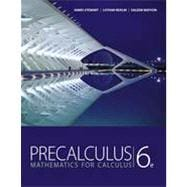# Precalculus : Mathematics for Calculus

• ISBN13:

• ISBN10:

## 0840068077

• Edition: 6th
• Format: Hardcover
• Publisher: Cengage Learning

Note: Supplemental materials are not guaranteed with Rental or Used book purchases.

Purchase Benefits

•Free Shipping On Orders Over \$35!
Your order must be \$35 or more to qualify for free economy shipping. Bulk sales, PO's, Marketplace items, eBooks and apparel do not qualify for this offer.
•Get Rewarded for Ordering Your Textbooks! Enroll Now
•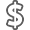We Buy This Book Back!
In-Store Credit: \$2.10
Check/Direct Deposit: \$2.00
PayPal: \$2.00
List Price: \$299.99 Save up to \$288.89
• Rent Book \$11.10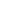Free Shipping

TERM
PRICE
DUE
IN STOCK USUALLY SHIPS IN 24 HOURS.
*This item is part of an exclusive publisher rental program and requires an additional convenience fee. This fee will be reflected in the shopping cart.

### Supplemental Materials

What is included with this book?

• The Used, Rental and eBook copies of this book are not guaranteed to include any supplemental materials. Typically, only the book itself is included. This is true even if the title states it includes any access cards, study guides, lab manuals, CDs, etc.

### Summary

This best selling author team explains concepts simply and clearly, without glossing over difficult points. Problem solving and mathematical modeling are introduced early and reinforced throughout, providing students with a solid foundation in the principles of mathematical thinking. Comprehensive and evenly paced, the book provides complete coverage of the function concept, and integrates a significant amount of graphing calculator material to help students develop insight into mathematical ideas. The authors' attention to detail and clarity, the same as found in James Stewart's market-leading Calculus text, is what makes this text the market leader.

 Fundamentals Chapter Overview Real Numbers Exponents and Radicals Algebraic Expressions Fractional Expressions Equations Modeling with Equations Inequalities Coordinate Geometry Solving Equations and Inequalities Graphically Lines Modeling Variation Functions Chapter Overview What Is a Function? Graphs of Functions Getting Information from the Graph of a Function Average Rate of Change of a Function Transformations of Functions Combining Functions One-to-One Functions and Their Inverses Review TeSt. Focus on Modeling: Functions as Models Polynomial And Rational Functions Chapter Overview Quadratic Functions and Models Polynomial Functions and Their Graphs Dividing Polynomials Real Zeros of Polynomials Complex Numbers Complex Zeros and the Fundamental Theorem of Algebra Rational Functions Review TeSt. Focus on Modeling: Fitting Polynomial Curves to Data Exponential And Logarithmic Functions Chapter Overview Exponential Functions The Natural Exponential Function Logarithmic Functions Laws of Logarithms Exponential and Logarithmic Equations Modeling with Exponential and Logarithmic Functions Review TeSt. Focus on Modeling: Fitting Exponential and Power Curves to Data Cumulative Review Test: Chapters 2, 3, and 4 Trigonometric Functions: Unit Circle Approach Chapter Overview The Unit Circle Trigonometric Functions of Real Numbers Trigonometric Graphs More Trigonometric Graphs Inverse Trigonometric Functions and Their Graphs Modeling Harmonic Motion Review TeSt. Focus on Modeling: Fitting Sinusoidal Curves to Data Trigonometric Functions: Right Triangle Approach Chapter Overview Angle Measure Trigonometry of Right Triangles Trigonometric Functions of Angles Inverse Trigonometric Functions and Triangles The Law of Sines The Law of Cosines Review TeSt. Focus on Modeling: Surveying Analytic Trigonometry Chapter Overview Trigonometric Identities Addition and Subtraction Formulas Double-Angle, Half-Angle, and Sum-Product Identities Basic Trigonometric Equations More Trigonometric Equations Review TeSt. Focus on Modeling: Traveling and Standing Waves Cumulative Review Test: Chapters 5, 6, and 7 Polar Coordinates And Parametric Equations Chapter Overview Polar Coordinates Graphs of Polar Equations Polar Form of Complex Numbers DeMoivre's Theorem Plane Curves and Parametric Equations Review TeSt. Focus on Modeling: The Path of a Projectile Vectors In Two And Three Dimensions Chapter Overview Vectors in Two Dimensions The Dot Product Three -Dimensional Coordinate Geometry Vectors in Three Dimensions The Cross Product Equations of Lines and Planes Review TeSt. Focus on Modeling: Vector Fields Cumulative Review Test: Chapters 8 and 9 Systems Of Equations And Inequalities Chapter Overview Systems of Linear Equations in Two Variables Systems of Linear Equations in Several Variables Systems of Linear Equations: Matrices The Algebra of Matrices Inverses of Matrices and Matrix Equations Determinants and Cramer's Rule Partial Fractions Systems of Non-Linear Equations Systems of Inequalities Review TeSt. Focus on Modeling: Linear Programming Analytic Geometry Overview Parabolas Ellipses Hyperbolas Shifted Conics Rotation of Axes Polar Equations of Conics Review TeSt. Focus on Modeling: Conics in Architecture Cumulative Review Test: Chapters 10 and 11 Sequences And Series Chapter Overview Sequences and Summation Notation Arithmetic Sequences Geometric Sequences Mathematics of Finance Mathematical Induction The Binomial Theorem Review TeSt. Focus on Modeling: Difference Equations Limits: A Preview Of Calculu.S Chapter Overview Finding Limits Numerically and Graphically Finding Limits Algebraically Tangent Lines and Derivatives Limits at Infinity: Limits of Sequences Areas Review TeSt. Appendix: Calculators and Calculations Table of Contents provided by Publisher. All Rights Reserved.

### Customer Reviews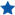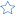Basically algebra June 24, 2014
"While it says precalculus, this book is mostly what was covered in my Algebra II class in high school, where I didn't have to pay tuition."Flag Review
Please provide a brief explanation for why you are flagging this review:
Your submission has been received. We will inspect this review as soon as possible. Thank you for your input!Decent at best October 17, 2011
This book has been hard for me to understand, however I have been giving it more time and things are improving. This book just takes more time to understand the concepts, which isn't uncommon for college math books.Flag Review
Please provide a brief explanation for why you are flagging this review:
Your submission has been received. We will inspect this review as soon as possible. Thank you for your input!
Precalculus : Mathematics for Calculus: 3 out of 5 stars based on 2 user reviews.